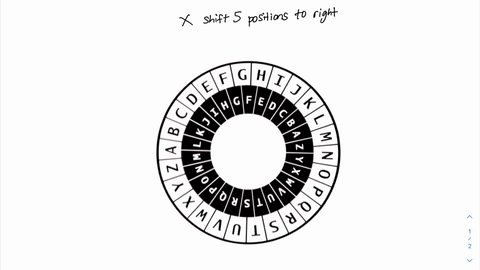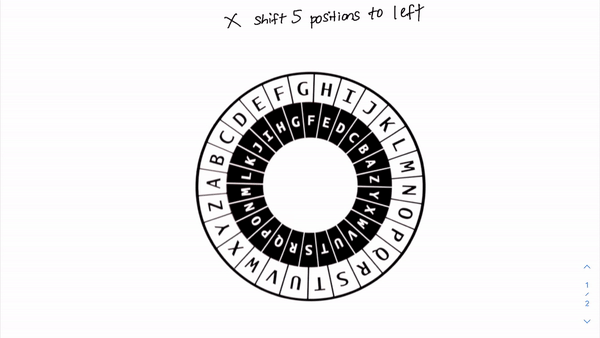# [Cybersecurity Series] Caeser Cipher

## Simplest way to encrypt & decrypt ASCII texts

So, here’s the SECRET to a good Caeser salad — add some avocados. But this is not a food blog, so let’s talk about some ancient ciphers used in cryptography instead.

# Substitution Cipher

A substitution cipher protect the data confidentiality by swapping the original characters to another random character in any text data.

For example, consider Alice sending text ‘ABC’ to Bob. Using any substitution cipher, Alice can encrypt the plaintext ‘ABC’ into a ciphertext like ‘DUA’ before sending it to Bob. Here, the character ‘A’ is replaced by ‘D’; ‘B’ is replaced by ‘U’ and C is replaced by ‘A’. Thus, we can say that ‘A’ is substituted by ‘D’. In this way, the confidentiality of the data in transit can be protected.

Plaintext (P) is the original message; ciphertext (C) is the ‘garbled message’. If we send a data in plaintext, the man-in-the-middle can intercept and reads this data. If we send the same data in ciphertext, the man-in-the-middle can still intercept the message but all they see is some scrambled text that does not represent the original text.

# Caeser cipher

Caeser cipher is a classic cryptography method used to encrypt plaintext (P) to ciphertext (C), or to decrypt ciphertext (C ) to plaintext (P). Caeser cipher is a special type of substitution cipher.

Caeser cipher works based on SHIFT operations. The shift here means it can swap an character with any character to the left or to the right of the target character in the alphabects list. For example, ‘B’ can be replaced with ‘A’ which is to its left, or replaced by ‘C’ which is to its right.

One important parameter of any cipher is the encryption/decryption KEY. The KEY of Caeser cipher is the magnitude of shift. This simply means ‘how many positions’ the character need to move to change from plaintext to ciphertext. For example,

`Example1========Plaintext:  ABCCiphertext: BCDA is shifted into B. This is a right shift, by 1 position. B is shifted into C. This is a right shift, by 1 position.C is shifted into D. This is a right shift, by 1 position. The KEY here is +1 to the right.Example2========Plaintext:   AVOCiphertext:  CXQA is shifted into C. A->B->C so this is a right shift, by 2 positionV is shifted into X. V->W->X so this is a right shift, by 2 positionO is shifted into Q. O->P->Q so this is a right shift, by 2 positionThe KEY here is +2 to the right.`

The alphabet list is cyclic, meaning that they are wrapped around as a rotor (instead of an array).Caeser Cipher, shift +5; to the right. Right also means clockwise. Here, ‘X’ is transposed to ‘C’Caeser Cipher, shift -5; to the left. Left also means counter-clockwise. Here, ‘X’ is transpose to ‘S’

# How to ‘decode’ Caeser Cipher

Given a plaintext (P) that is encrypted with Caeser cipher, you can easily find the corresponding Ciphertext (C) if you know the KEY. There are 3 possible common scenarios:

(A) find the C if we have the {P, key}

(B) find the P if we have the {C, key}

(C) find the P if we only have the {C}

There are 2 ways to do this, if you want to go fast use Method 1; if you want to go far, use Method 2.

## Method 1 — Using a Caesar Cipher Calculator

You can easily and quickly decrypt with Caeser Cipher using the Cryptii Cipher en/decoder. Remember the rules “busy does not mean more productive”. In CTF, always use any online tools that can help you crack the code (because every seconds count!).

Here’s a guided video on how to use Cryptii to encrypt and decrypt text data.

## Method 2 — Build our own shift table (manual)

(A) Find the C if we have the {P, key}

Here, we manually count to the new character by the value (key) from the original character. For example:

`Plaintext: bdLlnbbCiphertext: ?Key: +17 to the rightStep1: Build a +17 plaintext to ciphertext mapping table (see video below)Step2: Replace the character in plaintext column with the character in the ciphertext column`

(B) Find the P if we have the {C, key}

`Plaintext: ?Ciphertext: successKey: +17 to the rightStep1: Build a +17 plaintext to ciphertext mapping table Step2: Replace the character in cipher text column with the character in the plaintext column`

(C) Find the P if we only have the {C} but no key

`Plaintext: ?Ciphertext: Cxq ku c jcpfuqog dqaKey: ?Step1: Build a plaintext to ciphertext mapping table Step2: The trick here is to guess the key based on linguistic rules. In English language, only two words have a single character = that is 'a' and 'I'. For example, I am a handsome boy. Notice that the word 'I' and 'a' only have 1 syllable, or character. Step3. Based on the 'logic' in step2, we guess that the 'c' in [Cxq ku c jcpfuqog dqa] most likely to be 'I' or 'a'. So, we build two shift table, 1 for 'c->I' and 1 for 'c->a'Step4, Replace the character in ciphertext column with the character in the plaintext column. Trial and error. Start with 'c->i' first. Then check if the output make sense (is it in English?). If so, that is the correct key shift. Else, try again with 'c->a'.`

I’m just a little boy, lost in the tech world. But remember, love is a riddle, and life with tech is more amazing than ever